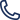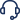530-820-3390 530-820-3390Support

## How to write multiple data in an excel sheet in an iterative manner?

You can write excel data in an iterative manner with the help of for loop.

Lets say we have a sample excel file as shown below in which you want to enter data in an iterative manner.

Assuming you want to enter some value in the count column and the expected output in the excel sheet will be something like below:

Step 2: Set a variable with sequence number to iterate one by one and to initialize the variable.

Step 3: Get the number of rows in the excel sheet which will be used in the for loop as the end index.

Step 4: Start for loop with startindex as 0 and end index as total number of rows in the excel sheet. Here it will be \${rowcount}

Step 5: Set the sequence number variable again to be iterated inside for loop.

Step 6: Define a variable and use setsetvalue function to set the data in the excel sheet.

Here, data is being written in the count column of the excel sheet one by one starting from the first index. \${count} refers to the current sequence number coming from the sequence number function as the input data and the next \${count} refers to the index of the excel sheet that at which index this data will be entered.

Syntax for getcellvalue is Key=Input Value.Index

Step 7: This step will mark the end of for loop.

So the complete test case will look as below:

To know more about the specific functions used in the above use case please refer this link.